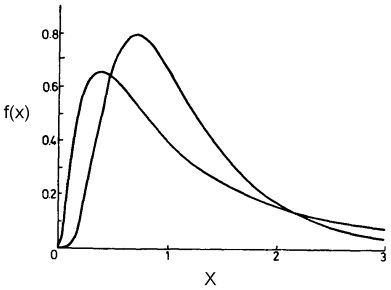The Rocscience International Conference 2021 Proceedings are now available. Read Now

# Lognormal Distribution

If a random variable has a Lognormal distribution, then its natural logarithm has a Normal distribution. This is the meaning of the term Lognormal.

Like the Exponential distribution, the Lognormal distribution can only be used for variables which are always positive.

A Lognormal distribution can be useful for modeling variables such as cohesion, for example, which may have a large peak in the distribution near zero and then taper off gradually for larger values.Lognormal probability density functions